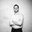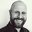Question-and-Answer Resource for the Building Energy Modeling Community
Get s tarted with the Help page

# WindowMaterial:Gas Type, Calculations, and PropertiesI am attempting to manually calculate the U-Value of a glazing unit in EnergyPlus without using the Envelope Summary Table output:meter. I noticed that when building a construction with WindowMaterial:Gas you can simply define the gas thickness and gas type (air, argon, xenon, krypton, and custom).

My question is, how does EnergyPlus calculate the gap gas, what are the equations, and what are the properties of the gases used?

Does EnergyPlus simulate them as a lumped conduction or does it calculate the convective heat transfer of that gap? I thought I would be able to find material property definitions and how they are used in EnergyPlus from the Engineering and Input/Output Reference Manuals but have not been able to find anything on gap calculations.

In section 6.8.15 Equivalent Layer Fenestration Model of the Engineering Reference Manual it's stated that:

  The convective heat transfer coefficient in a gap is calculated depending on the spacing, the temperature of the layers and the fill gas properties.


However, it doesn't provide equations. Maybe I'm looking in the wrong spot. Can someone point me in the right direction?

Justin

edit retag close merge delete

Sort by » oldest newest most votedThe window heat balance equations are the Engineering Reference.

The section on the equivalent layer thermal model is here.

more